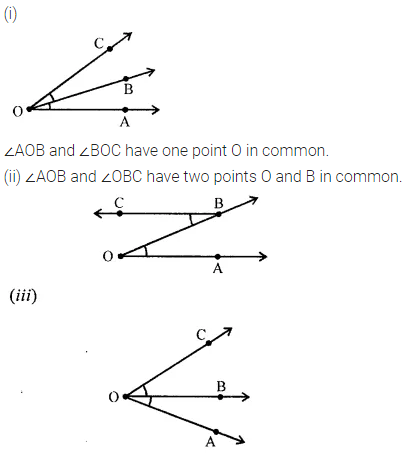ML Aggarwal Class 6 Solutions Chapter 10 Basic Geometrical Concept Ex 10.2 for ICSE Understanding Mathematics acts as the best resource during your learning and helps you score well in your exams.

## ML Aggarwal Class 6 Solutions for ICSE Maths Chapter 10 Basic Geometrical Concept Ex 10.2

Question 1.
How many angles are shown in the following figure? Name them.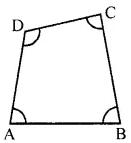Solution: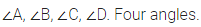Question 2.
In the given figure, name the point(s)
(i) In the interior of ∠DOE
(ii) In the exterior of ∠EOF
(iii) On ∠EOF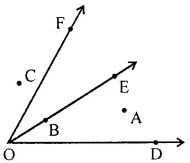Solution: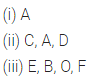Question 3.
Draw rough diagrams of two angles such that they have
(i) One point in common.
(ii) Two points in common.
(iii) One ray in common.
Solution: